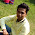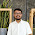## Sunday, 20 August 2017

### Program For Single Columnar Transposition

Program:

import java.util.*;
class simpleColumnar{
public static void main(String sap[]){
Scanner sc = new Scanner(System.in);

System.out.print("\nEnter plaintext(enter in lower case): ");
String message = sc.next();
System.out.print("\nEnter key in numbers: ");
String key = sc.next();

/* columnCount would keep track of columns */
int columnCount = key.length();

/* rowCount will keep of track of rows...no of rows = (plaintextlength + keylength) / keylength */
int rowCount = (message.length()+columnCount)/columnCount;

/*plainText and cipherText would be array containing ASCII values for respective alphabets */
int plainText[][] = new int[rowCount][columnCount];
int cipherText[][] = new int[rowCount][columnCount];

/*Encryption Process*/
System.out.print("\n-----Encryption-----\n");
cipherText = encrypt(plainText, cipherText, message, rowCount, columnCount, key);

// prepare final string
String ct = "";
for(int i=0; i<columnCount; i++)
{
for(int j=0; j<rowCount; j++)
{
if(cipherText[j][i] == 0)
ct = ct + 'x';
else{
ct = ct + (char)cipherText[j][i];
}
}
}
System.out.print("\nCipher Text: " + ct);

/*Decryption Process*/
System.out.print("\n\n\n-----Decryption-----\n");

plainText = decrypt(plainText, cipherText, ct, rowCount, columnCount, key);

// prepare final string
String pt = "";
for(int i=0; i<rowCount; i++)
{
for(int j=0; j<columnCount; j++)
{
if(plainText[i][j] == 0)
pt = pt + "";
else{
pt = pt + (char)plainText[i][j];
}
}
}
System.out.print("\nPlain Text: " + pt);

System.out.println();
}

static int[][] encrypt(int plainText[][], int cipherText[][], String message, int rowCount, int                                                                                                           columnCount, String key){
int i,j;
int k=0;

/* here array would be filled row by row  */
for(i=0; i<rowCount; i++)
{
for(j=0; j<columnCount; j++)
{
/* terminating condition...as string length can be smaller than 2-D array */
if(k < message.length())
{
/* respective ASCII characters would be placed */
plainText[i][j] = (int)message.charAt(k);
k++;
}
else
{
break;
}
}
}

/* here array would be filled according to the key column by column */
for(i=0; i<columnCount; i++)
{
/* currentCol would have current column number i.e. to be read...as there would be ASCII value stored in key so we would subtract it by 48 so that we can get the original number...and -1 would be subtract as array position starts from 0*/
int currentCol= ( (int)key.charAt(i) - 48 ) -1;
for(j=0; j<rowCount; j++)
{
cipherText[j][i] = plainText[j][currentCol];
}

}

System.out.print("Cipher Array(read column by column): \n");
for(i=0;i<rowCount;i++){
for(j=0;j<columnCount;j++){
System.out.print((char)cipherText[i][j]+"\t");
}
System.out.println();
}

return cipherText;
}

static int[][] decrypt(int plainText[][], int cipherText[][], String message, int rowCount, int                                                                                                          columnCount, String key){
int i,j;
int k=0;

for(i=0; i<columnCount; i++)
{
int currentCol= ( (int)key.charAt(i) - 48 ) -1;
for(j=0; j<rowCount; j++)
{
plainText[j][currentCol] = cipherText[j][i];
}
}

System.out.print("Plain Array(read row by row): \n");
for(i=0;i<rowCount;i++){
for(j=0;j<columnCount;j++){
System.out.print((char)plainText[i][j]+"\t");
}
System.out.println();
}

return plainText;
}
}

Output:

Enter plaintext(enter in lower case): networksecurity

Enter key in numbers: 31452

-----Encryption-----
t       n       w       o       e
s       r       e       c       k
i       u       t       y       r

Cipher Text: tsixnruxwetxocyxekrx

-----Decryption-----
n       e       t       w       o
r       k       s       e       c
u       r       i       t       y

Plain Text: networksecurity

1.1.Thanks Bro...

2.Thanks for giving single columnar transposition program which improves my knowledge a lot. Keep updating...
Best Online Software Training Institute | Core Java Training

3.what we need to do if we want that key should accept letters digits also?

4.myTectra Placement Portal is a Web based portal brings Potentials Employers and myTectra Candidates on a common platform for placement assistance

5.6.7.8.9.10.Really this is a very nice informative post. This is the good informative post for the Core Java freshers. Thank you for posting the information.

11.Hi I want to talk about one site fantastic best gambling sites there I spend all my free time there I spend all my free time and get a small income

12.Thank you for your post. This is excellent information. It is amazing and wonderful to visit your site.